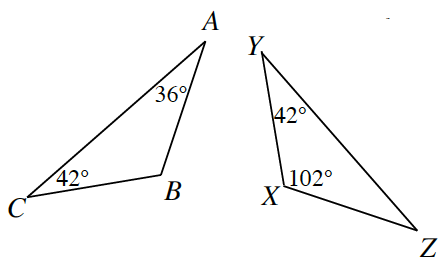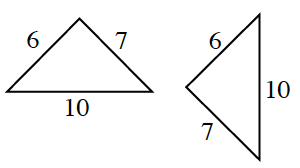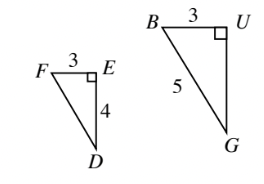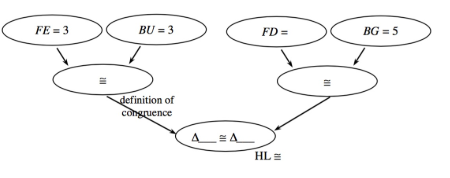### Home > GB8I > Chapter 7 Unit 8 > Lesson INT1: 7.2.3 > Problem7-107

7-107.

For each pair of triangles below, determine whether or not the triangles are congruent. If they are congruent, show your reasoning in a flowchart. If they are not congruent, explain why not.

1.Calculate the values of the missing angles. What do you notice?

Does $\text{AAA}$ guarantee triangle congruence?

1.Does $\text{SSS}$ guarantee triangle congruence?

1.These are both right triangles. Use the Pythagorean Theorem to calculate the lengths of the missing legs.

Complete the proof below.